Select Page

# MCQ in Hindi Vector Algebra Solutions for CBSE Maths 12 Science

MCQ in Hindi Vector Algebra Solutions for CBSE Maths 12 Science to enable students to get Solutions in a narrative video format for the specific question.

Expert Teacher provides MCQ Vector Algebra Solutions for CBSE Maths 12 Science through Video Solutions in Hindi language. This video solution will be useful for students to understand how to write an answer in exam in order to score more marks. This teacher uses a narrative style for a question from Vector Algebra not only to explain the proper method of answering question, but deriving right answer too.

Please find the question below and view the Solution in a narrative video format.

Question:

Solution Video in Hindi:

You can select video Solutions from other languages also. Please check Solutions in ( English )

## Similar Questions from CBSE, 12th Science, Maths, Vector Algebra

Question 1 :  Find the sum of the vectors :(View Answer Video)

Question 2 :  Find the sum of the vectorsand(View Answer Video)

Question 3 :  Write a vector in the direction of the vectorthat has magnitude 9 units.   (View Answer Video)

Question 4 :  Ifandare two equal vectors, then write the value of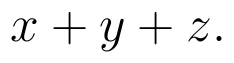(View Answer Video)

Question 5 : Find the value of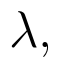if the points with position vectorsandare coplanar.  (View Answer Video)

### Matrices

Question 1 : Find the value of z,  from the equation:(View Answer Video)

Question 2 : If, then find "a". (View Answer Video)

Question 3 : Given,, find the value of x. (View Answer Video)

Question 4 : If, write the value of"x". (View Answer Video)

Question 5 : Find the value of X, ifand. (View Answer Video)

### Continuity and Differentiability

Question 1 : Differentiate the functionwith respect to x. (View Answer Video)

Question 2 : Differentiate w.r.t.x the function. (View Answer Video)

Question 3 : Findfor the function. (View Answer Video)

Question 4 : If x and y are connected parametrically by the equation, without eliminating the parameter, find. (View Answer Video)

Question 5 : Findfor the function. (View Answer Video)

### Probability

Question 1 : If P(A)=0.8, P(B)=0.5 and=0.4, find. (View Answer Video)

Question 2 : If E and F are two events such that,, Find P(not E and not F). (View Answer Video)

Question 3 : Compute P(A/B) if P(B) =0.5 and P(A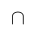B)=0.32. (View Answer Video)

Question 4 : If P(A)=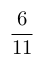, P(B)=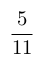and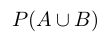=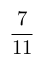, find. (View Answer Video)